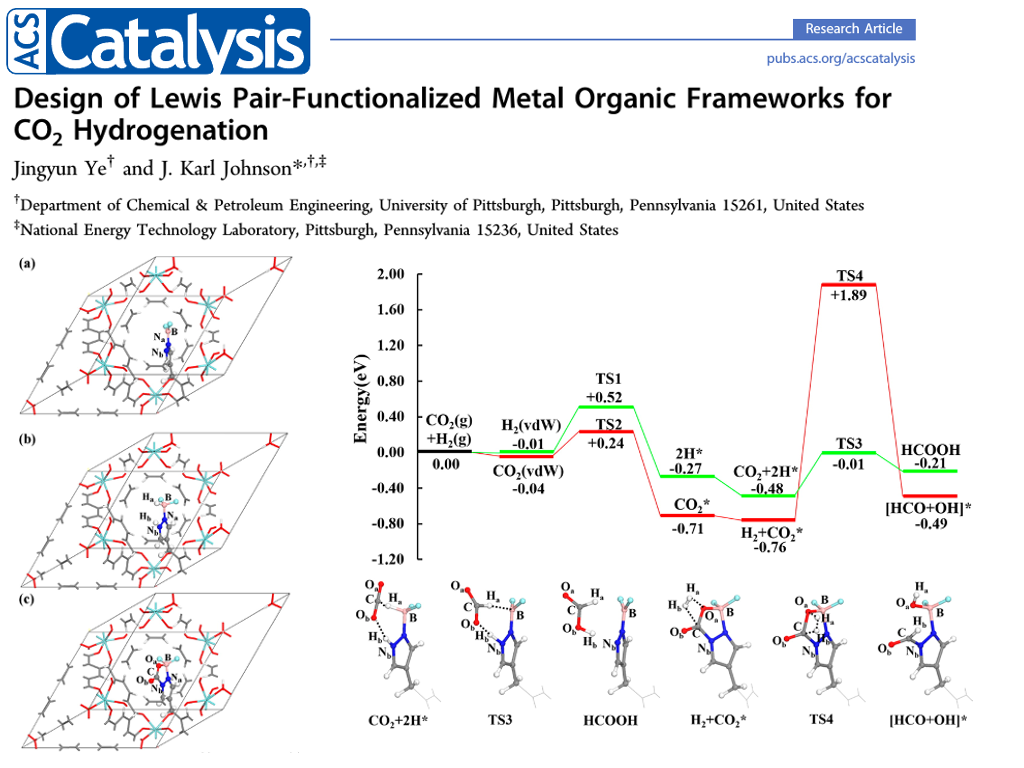#CP2K Open Source Molecular Dynamics

### Site Tools

exercises:common:geo_opt

# Geometry Optimization

## Introduction

Geometry optimization is a process of changing the system's geometry (the nuclear coordinates and potentially the lattice vectors) to minimize the total energy of the systems.

Potential energy surface describes the energy of a system, especially a collection of atoms, in terms of certain parameters, normally the positions of the atoms.

Consider a potential energy surface (PES) as below, the goal is to find the global (or local) minimum, such as the minimum for reactant or product. The commonly-used algorithms include Conjugate gradient method , Quasi-Newton method and its variant BFGS method.

None of these methods guarantee to find the global minimum.

The BFGS method is more efficient when the initial guess is not far from the minimum.

Mathematically, the minimum should fulfill two requirements:

1. The gradient should be zero, $\frac{dE}{dr} = 0$

2. The sign of Hessian should be all positive, $\frac{d^2E}{dr^2} > 0$

Gradient: the first derivative of the energy with respect to geometry, also termed the Force, $f = -\frac{dE}{dr}$.

Hessian: the second derivative of the energy with respect to geometry, $\frac{d^2E}{dr^2}$

To ensure these requirements, one should perform Vibrational Analysis to examine the eigenvalues of the Hessian. If there are some negative values, it means this point is not the minimum.

## Exercies

In this exercise, you will perform geometry optimization using DFT. See GEO_OPT

Note the different RUN_TYPE and the changed PROJECT name. The latter is not strictly necessary but recommended since CP2K automatically creates additional files using this project name as a prefix.

H2O.inp
&GLOBAL
PROJECT H2O
RUN_TYPE GEO_OPT
PRINT_LEVEL MEDIUM
&END GLOBAL

&MOTION
&GEO_OPT
MAX_ITER 3000
OPTIMIZER BFGS    #Most efficient minimizer, but only for 'small' systems
&END GEO_OPT
&END MOTION

&FORCE_EVAL
METHOD Quickstep              ! Electronic structure method (DFT,...)
&DFT
BASIS_SET_FILE_NAME  BASIS_MOLOPT
POTENTIAL_FILE_NAME  POTENTIAL

&POISSON                    ! Solver requested for non periodic calculations
PERIODIC NONE
PSOLVER  WAVELET          ! Type of solver
&END POISSON
&SCF                        ! Parameters controlling the convergence of the scf. This section should not be changed.
SCF_GUESS ATOMIC
EPS_SCF 1.0E-6
MAX_SCF 300
&END SCF
&XC                        ! Parameters needed to compute the electronic exchange potential
&XC_FUNCTIONAL PBE
&END XC_FUNCTIONAL
&END XC
&END DFT

&SUBSYS
&CELL
ABC 10 10 10
PERIODIC NONE              ! Non periodic calculations. That's why the POISSON section is needed
&END CELL
&TOPOLOGY                    ! Section used to center the atomic coordinates in the given box. Useful for big molecules
&CENTER_COORDINATES
&END
COORD_FILE_FORMAT xyz
COORD_FILE_NAME  ./H2O.xyz
&END
&KIND H
ELEMENT H
BASIS_SET DZVP-MOLOPT-GTH
POTENTIAL GTH-PBE-q1
&END KIND
&KIND O
ELEMENT C
BASIS_SET DZVP-MOLOPT-GTH
POTENTIAL GTH-PBE-q6
&END KIND
&END SUBSYS
&END FORCE_EVAL
H2O.xyz

3
Water
O 5 5.00000 5.11779
H 5 5.75545 4.52884
H 5 4.24455 4.52884

You can also directly open an XYZ file in VMD to visualize it:

$vmd H2O.xyz After running this, you will have the following files: $ ls H2O*
H2O-1.restart        H2O-1.restart.bak-3  H2O.out          H2O-RESTART.wfn.bak-1
H2O-1.restart.bak-1  H2O-BFGS.Hessian     H2O-pos-1.xyz    H2O-RESTART.wfn.bak-2
H2O-1.restart.bak-2  H2O.inp              H2O-RESTART.wfn  H2O-RESTART.wfn.bak-3

Take a look at the output file, especially the following section (repeated the number of cycles it took to reach convergence):

 --------  Informations at step =     1 ------------
Optimization Method        =                 BFGS
Total Energy               =       -14.9417142787
Real energy change         =        -0.1955604816
Predicted change in energy =        -0.1885432833
Scaling factor             =         0.0000000000
Step size                  =         0.2677976891
Decrease in energy         =                  YES
Used time                  =               19.018

Convergence check :
Max. step size             =         0.2677976891
Conv. limit for step size  =         0.0030000000
Convergence in step size   =                   NO
RMS step size              =         0.1458070233
Conv. limit for RMS step   =         0.0015000000
Convergence in RMS step    =                   NO
Conv. limit for gradients  =         0.0004500000
Conv. limit for RMS grad.  =         0.0003000000
---------------------------------------------------
Geometry optimization has been widely used in surface science and computational catalysis. Based on electronic structure theory or force fields, the structures are optimized under 0 K to calculate the potential energy. To obtain the Gibbs free energy, one can use $G = E_{DFT} + ZPE - TS$, where the latter two terms can be estimated by the Vibrational Analysis .Jingyun Ye & J. Karl Johnson; 2015; Design of Lewis Pair-Functionalized Metal Organic Frameworks for CO2 Hydrogenation ACS Catal. 5: 2921−2928